# Create a Five Star Rating System

This post will guide you how to create a five star rating system in your current worksheet in excel. How do I change the five points system to five star rating in excel. How to use the conditional formatting function to create a five star rating system in excel.

## Create a Five Star Rating System

The five star rating system is a very useful way of visualizing rating like you see on Amazon site.

For example, you have a list of data in your current worksheet and it contains rating values in each cell in rating column, and you want to create a five star rating system in your worksheet, just do the following steps:

#1 select the range of cells (C1:G1) and merging it as one cell, then type “Star Rating” text in this cell.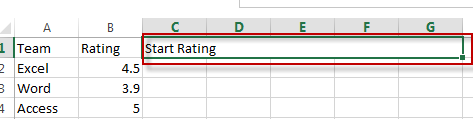#2 type the numbers from 1 to 5 into each cells of range H1:L1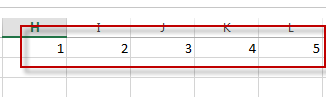#3 type the following formula into the formula box of Cell C2, then drag the Auto Fill Handle over other cells (C2:G3) to apply this formula.

`=IF(\$B2>=H\$1,1,IF(INT(\$B2)=H\$1-1,MOD(\$B2,1),0))`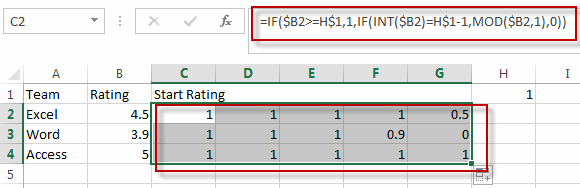#4 select the range of star rating (C2:G3), go to HOME tab, click Conditional Formatting command under Styles group, then select New Rule from the drop down list. The New Formatting Rule dialog will appear.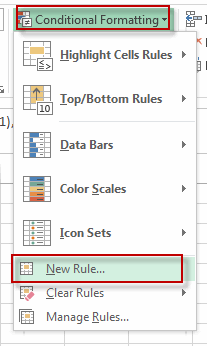#5 click Format all cells based on their values from Select a Rule Type list. Choose Icon Sets from the Format Style, select 3 stars icon from Icon Style. Check Show Icon Only box. Then set a full star is shown if the value is greater than or equal to 1, and a half star if the value is greater than or equal to 0.5.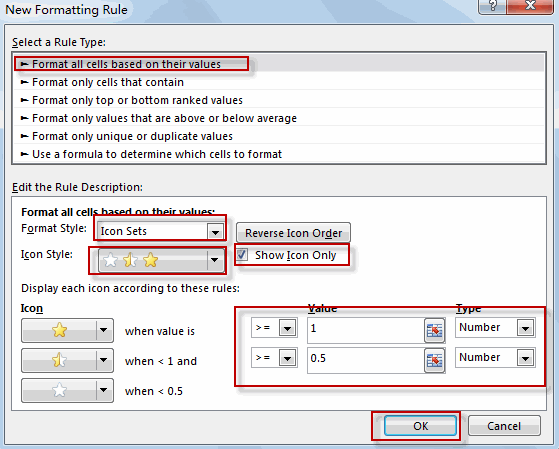#6 let’s see the result.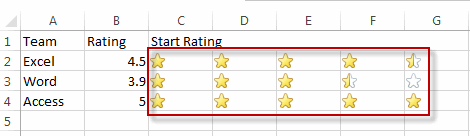### Related Functions

• Excel IF function
The Excel IF function perform a logical test to return one value if the condition is TRUE and return another value if the condition is FALSE. The IF function is a build-in function in Microsoft Excel and it is categorized as a Logical Function.The syntax of the IF function is as below:= IF (condition, [true_value], [false_value])….
• Excel MOD function
he Excel MOD function returns the remainder of two numbers after division. So you can use the MOD function to get the remainder after a number is divided by a divisor in Excel. The syntax of the MOD function is as below:=MOD (number, divisor)….
• Excel INT function
The Excel INT function returns the integer portion of a given number. And it will rounds a given number down to the nearest integer.The syntax of the INT function is as below:= INT (number)…